# Bohr model of Sodium atom - How to draw Sodium(Na) Bohr-Rutherford diagram?

Home  > Chemistry Article > Sodium Bohr model

The Bohr Model of Sodium(Na) has a nucleus that contains 12 neutrons and 11 protons. This nucleus is surrounded by three-electron shells named K-shell, L-shell, and M-shell. The outermost shell in the Bohr diagram of Sodium contains only 1 electron that also called valence electron.

 Name Sodium Bohr Model Number of neutrons 12 Number of protons 11 Number of electrons 11 Total electron shells 3 Electron in the First shell(K) 2 Electrons in the Second shell(L) 8 Electrons in the Third shell(M) 1 Total valence electrons in Sodium 1
Page Contents

## How to draw Bohr Model of Sodium(Na)?

Bohr model describes the visual representation of orbiting electrons around the small nucleus. It used different electron shells such as K, L, M, N…so on. These shells hold a specific number of electrons, the electron shell which is closest to the nucleus has less energy and the electron shell which is farthest from the nucleus has more energy.

Bohr diagram is very interesting and easy to draw. Here, we will draw the Bohr diagram of the Sodium atom with some simple steps.

## Steps to draw the Bohr Model of Sodium atom

1. Find the number of protons, electrons, and neutrons in the Sodium atom

Protons are the positively charged particles and neutrons are the uncharged particles, both these are constituents of the atom nuclei. Electrons are the negatively charged particles that orbit the nucleus of an atom

To find the number of protons an atom contains, just look at their atomic number.

If the atomic number of atom is 15, then proton will also be 15, if atomic number is 16, then proton will also be 16.

So, the atomic number for Sodium is 11, hence, the number of protons in the Sodium atom is also 11.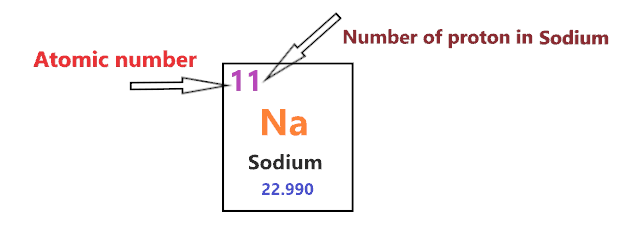Now, to determine the number of neutrons in an atom, use this formula.

⇒ Number of neutrons in atom = Atomic mass of the atom(rounded to the nearest whole number) – Number of proton in an atom

For example, An atom have 13.99 atomic mass and 6 protons.

Then, to find the number of neutron, round the atomic mass to the near whole number, so, atomic mass 13.99 round to 14.

= (14 – 6 protons) = 8 number of neutrons in the atom

Now, To get the number of neutrons in a Sodium atom, look at its atomic mass which is 22.990 rounded to 23, and the number of protons in Sodium is 11.

∴ Hence, the number of neutrons in Sodium atom = (23 – 11) = 12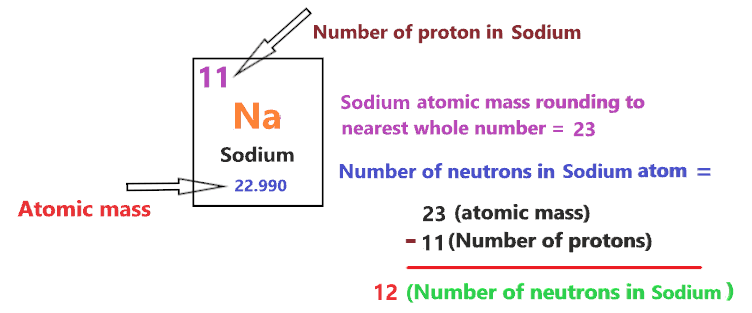It should be noted that “The number of electrons in a neutral atom is equal to the number of protons”.

So, the Sodium atom is neutral, hence, its number of electrons will be equal to the number of protons which is 11 as we already discussed.

⇒ The number of electrons in Sodium atom = 11

⇒ The number of protons in Sodium atom = 11

⇒ The number of neutrons in Sodium atom = 12

2. Draw the nucleus of an atom

A nucleus is a dense and small region that contains the number of protons and neutrons of an atom.

In this step, we have to draw a small circle that consists of a number of protons and the number of neutrons of a Sodium atom.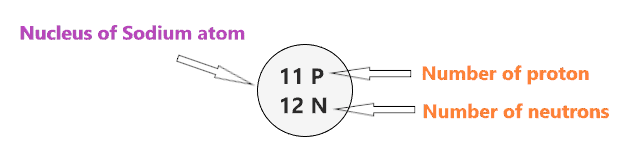3. Draw the First electron shell

“An electron shell may be thought of as an orbit followed by electrons around an atom’s nucleus.”

The first electron shell is also called the K-shell, this is the closest shell to the nucleus of an atom and can hold a maximum of two electrons.

As we know, the Sodium atom has a total of 11 electrons. So, put two electrons from it, in the first shell, next to each other.We have successfully drawn the first shell of the Sodium atom that can hold 2 electrons. As Sodium atoms have a total of 11 electrons, and from 11 electrons we have used two electrons in the first shell.

∴ (11 – 2) = 9 electrons

Therefore, we are left with 9 electrons, let’s put them in the next shells of the Sodium atom.

4. Draw the Second electron shell

The second shell also called the L-shell that can hold a maximum of 8 electrons. This shell is drawn after the first electron shell.

In the second electron shell, the electrons are added one at a time, starting from the top position then going in a clockwise direction.

In second shell, electrons are added one at a time in clockwise direction as a clock position – 12 o’clock, 3 o’clock, 6 o’clock, 9 o’clock positions.Once you place the electrons one at a time to each of the four sides(Top – Right – Bottom – Left], start pairing or doubling them.

So, we have 9 remaining electrons of a sodium atom, and the 2nd shell can only hold a maximum of 8 electrons.

Therefore, put the 8 electrons of the Sodium atom in the 2nd electron shell, start from the top position, put electrons one at a time, and go in a clockwise direction(Top – Right – Bottom – Left). And, finally, pair them up.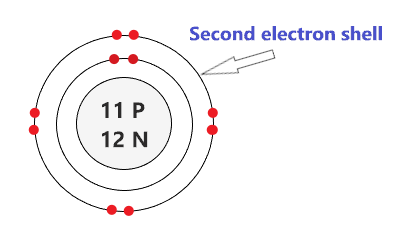As we have a total of 11 electrons for the sodium atom and we placed 2 electrons in the first shell and 8 electrons in the second shell, hence, we are left with only 1 electron more.

Let’s place it in the next shell.

5. Draw the Third electron shell

The third shell is also called the M-shell and it has the capacity to hold up to 18 electrons but, for the elements in the first few periods, the third shell only holds up to 8 electrons.

“The third shell can be considered to hold 8 or 18 electrons but in total the third shell can hold 18 electrons.”

For the first few elements, say, till atomic number 20 on the periodic table, the third shell holds up to 8 electrons.

⇒ For atomic number 20 or less than 20, the third shell can hold up to maximum of 8 electrons.

⇒ For the atomic number more than 20, say, atomic numbers 21, 22, 23, 24, 25, 26, 27,…….etc., the third shell is filled with more than 8 electrons and up to maximum of 18 electrons.

So, we have left with only 1 electron of a sodium atom, just place it at the top position of the third shell.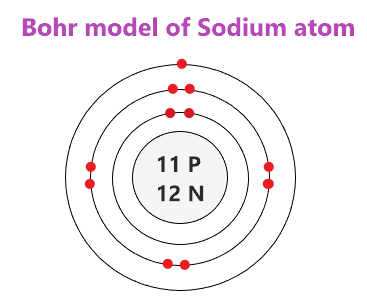### Bohr model of Sodium(Na)

That’s all, this is our Bohr model of the Sodium atom that contains 11 protons and 12 neutrons in the nucleus region, and 11 electrons are orbited around the nucleus, two electrons in the first shell, eight electrons in the second shell, and one electron in the third shell.

## Find Valence electron of Sodium through its Bohr diagram

Valence electrons are found in the outermost shell of an atom and they can take participate in the formation of a chemical bond. These electrons have more energy compare to the inner shell electrons.

From the Bohr diagram of an atom, we can easily find the number of valence electrons in an atom by looking at its outermost shell.

So, we have to find a valence electron in the Sodium atom, for this, look at its Bohr diagram.

Bohr’s diagram of Sodium has three electron shells (K, L, and M), the inner shell is K-shell and the outermost shell is M-shell.

Hence, the electrons found in the M-shell of the Sodium atom are its valence electrons because it is the outermost shell that also called the valence shell.

The M-shell or outer shell of the Sodium Bohr model contains only 1 electron, therefore, the number of the valence electrons in the Sodium atom is also 1.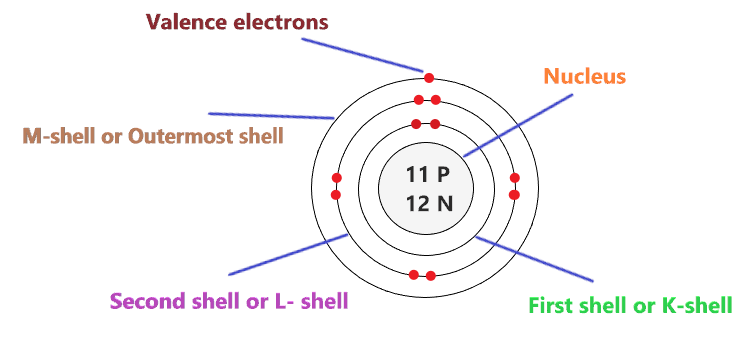## Electron dot diagram of a Sodium atom

Electron dot diagram also called lewis structure which represents the valence electrons of atoms.

As, from the Bohr diagram of Sodium, we got to know, it has only 1 valence electron.

So, just represent the 1 valence electrons around the Sodium atom as a dot.## The electron configuration of Sodium

Electron configuration is the distribution of electrons of an atom or molecule in atomic or molecule orbitals”

Sodium has an atomic number of 11 and it contains a total number of 11 electrons. From the Bohr model of Sodium, we know, 2 electrons are in the K-shell, 8 electrons are in the L-shell, and 1 electron is in the M-shell.

So, based on the shell, the electronic configuration of the Sodium atom is [2, 8, 1].

Or the electronic configuration of Sodium is 1s22s22p63s1 since it contains a total of 11 electrons, the first two-electron will go in 1s orbital, the next two in 2s orbital, the next six electrons in 2p orbital, and the remaining one electron will go in 3s orbital.

S orbital can hold maximum of 2 electrons and P orbital can hold maximum of 6 electrons.## FAQ

### How many electron shells a Sodium Bohr model contains?

Electron shell also called energy level, you can find the number of electron shells for an element by knowing its period number in the periodic table.

The elements or atoms in the first period of the periodic table have one energy level or one electron shell, same as, the elements in the second period have two energy levels or two-electron shells and so on…

So, the Sodium atom belongs to the 3rd Period in the periodic table, hence, the number of electron shells for the Bohr model of Sodium will be 3 (K-shell, L-shell, and M-shell).

### What is the outer shell of the Bohr diagram of the Sodium atom?

The outermost shell also called the valence shell, which contains valence electrons of the atom.

According to the Bohr diagram of Sodium, the outer shell is M-shell which contains only 1 valence electron.

## Properties of Sodium

1. Sodium appears as silvery-white metallic and highly reactive in nature.
2. It has a boiling point of 882.9 °C and a melting point of 97.7 °C.
3. It has a body-centered cubic crystal structure.
4. It is the seventh most abundant element on Earth.
5. Its electronegativity at the Pauling scale is 0.93.

## Summary

• The Bohr model of Sodium(Na) is drawn with three electron shells, the first shell contains 2 electrons, the second shell contains 8 electrons and the third shell contains 1 electron.
• Sodium is neutral and its atomic number is 11, hence, the number of protons and electrons available for its Bohr diagram is also 11.
• The number of neutrons for the Bohr diagram of Sodium can be found by subtracting the number of protons from the atomic mass(rounded to the nearest whole).
• The electron configuration of Sodium in terms of the shell is [2, 8, 1], or in normal form, it is [Ne] 3s1.
##### Subscribe to Blog via Email

Join 2 other subscribers

Share it...